### [原创]第十题 海风月影cm解题过程

2017-6-21 10:43
5079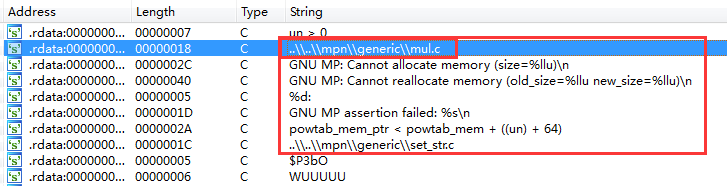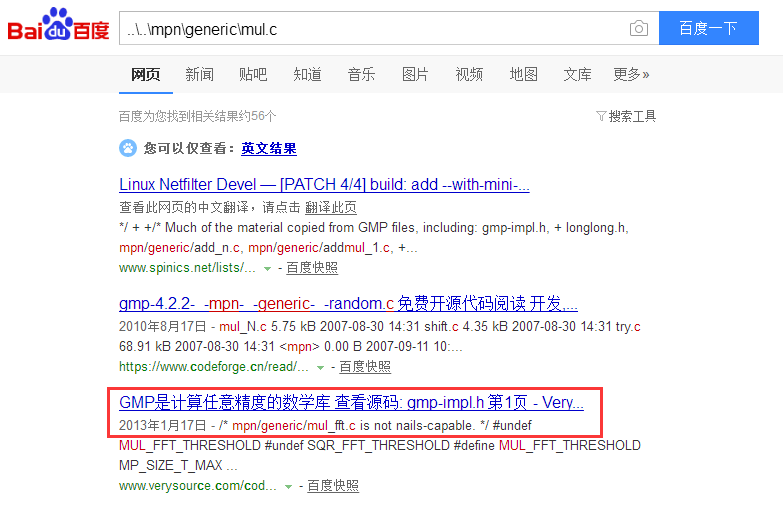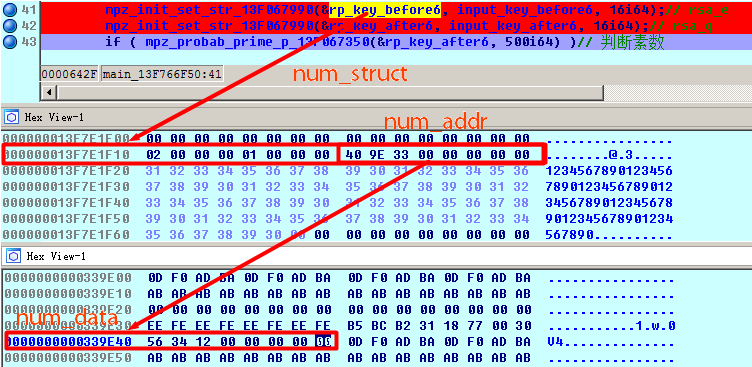```auto buf_rip;
auto buf_rdx;
auto buf_rax;
auto x1, x2, x3, x4, x5, x6;
auto y1, y2, y3, y4, y5, y6;
auto first;
first = 0;

for(x1=0; x1<16; x1++)
{
if(x1 <= 9)
{
y1 = 0x30 + x1;
}
else
{
y1 = 0x41 + x1 - 10;
}
for(x2=0; x2<16; x2++)
{
if(x2 <= 9)
{
y2 = 0x30 + x2;
}
else
{
y2 = 0x41 + x2 - 10;
}
for(x3=0; x3<16; x3++)
{
if(x3 <= 9)
{
y3 = 0x30 + x3;
}
else
{
y3 = 0x41 + x3 - 10;
}
for(x4=0; x4<16; x4++)
{
if(x4 <= 9)
{
y4 = 0x30 + x4;
}
else
{
y4 = 0x41 + x4 - 10;
}
for(x5=0; x5<16; x5++)
{
if(x5 <= 9)
{
y5 = 0x30 + x5;
}
else
{
y5 = 0x41 + x5 - 10;
}
for(x6=0; x6<16; x6++)
{
if(x6 <= 9)
{
y6 = 0x30 + x6;
}
else
{
y6 = 0x41 + x6 - 10;
}

if(first == 0)
{
first = 1;
continue;
}
buf_rip = GetRegValue("RIP");
buf_rip = buf_rip & 0xffffffffffff0000;
SetRegValue(buf_rip + 0x703B, "RIP");
RunTo(buf_rip + 0x704B);
GetDebuggerEvent(WFNE_SUSP, -1);

buf_rdx = GetRegValue("RDX");
PatchByte(buf_rdx + 63, y6);
PatchByte(buf_rdx + 62, y5);
PatchByte(buf_rdx + 61, y4);
PatchByte(buf_rdx + 60, y3);
PatchByte(buf_rdx + 59, y2);
PatchByte(buf_rdx + 58, y1);

RunTo(buf_rip + 0x7061);
GetDebuggerEvent(WFNE_SUSP, -1);

buf_rax = GetRegValue("RAX");
if(buf_rax != 0)Message("sucess!%x%x%x%x%x%x\r\n", y1, y2, y3, y4, y5, y6);

}
}
}
}
}
}```

```00000B（11）
00000D（13）
000011（17）
000013（19）
000017（23）
00001D（29）
00001F（31）
000025（37）
000029（41）
。。。。。。```

n = 0x6248BC3AB92A33B000FDB88568F19727F92F79EB68FF6AD73203EFD20A3E331BE941C7AA288095F33BC4B255FD983114D480EFFBEE2E313E6218A57F9CCC8189 = q * p  = 0x8DBDDE72E2E693B2AED5C769C0DCB3DA83534480A80E652FFE53544CD91A18C3 * 0xB182DE2AC2DB8735CF01B2AB4CC338C82E2806043302A3CE9EFA861DC36377C3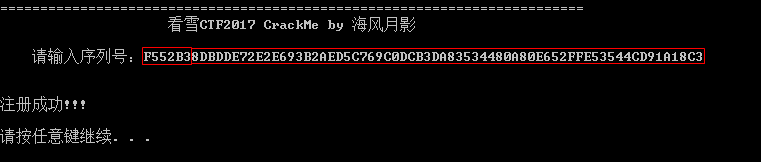2
0lhglhg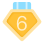1 2017-6-21 14:40
3
0
154位要分解多久呀？Ericky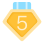6 2017-6-21 14:42
4
05
0风间仁 这个N是谁提交到那个网站的……ccfer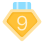16 2017-6-22 14:35
6
0